How elliptic curve cryptography works in TLS 1.3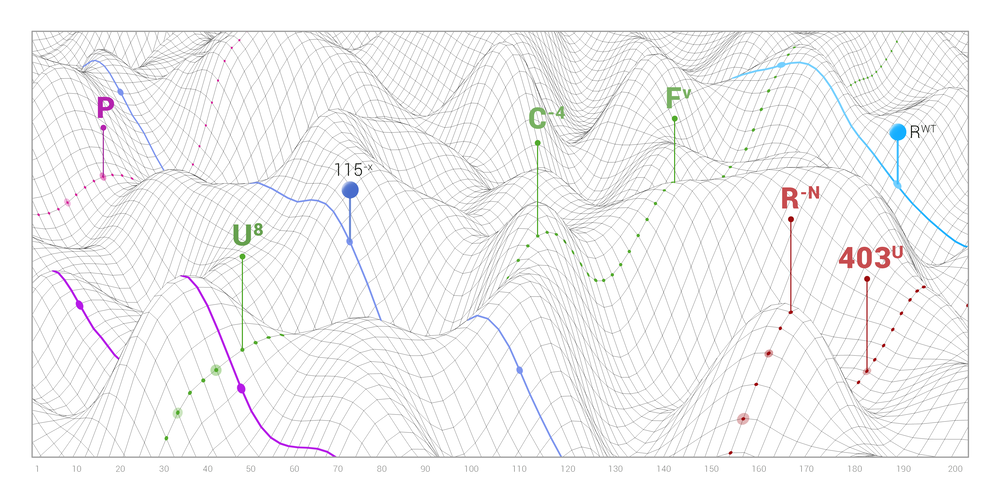In order to (somewhat) simplify the description process and tighten the volume of the article we are going to write, it is essential to make a significant remark and state the primary constraint right away — everything we are going to tell you today on the practical side of the problematics is viable only in terms of TLS 1.3. Meaning that while your ECDSA certificate would still work in TLS 1.2 if you wish it worked, providing backwards compatibility, the description of the actual handshake process, cipher suits and client-server benchmarks covers TLS 1.3 only. Of course, this does not relate to the mathematical description of algorithms behind modern encryption systems.

This article was written by neither a mathematician nor an engineer — although those helped to find a way around scary math and reviewed this article. Many thanks to Qrator Labs employees.

### (Elliptic Curve) Diffie-Hellman (Ephemeral)

The Diffie–Hellman legacy in the 21 century

Of course, this has started with neither Diffie nor Hellman. But to provide a correct timeline, we need to point out main dates and events.

There were several major personas in the development of modern cryptography. Most notably, Alan Turing and Claud Shannon both laid an incredible amount of work over the field of theory of computation and information theory as well as general cryptanalysis, and both Diffie and Hellman, are officially credited for coming up with the idea of public-key (or so-called asymmetric) cryptography (although it is known that in the UK there were made serious advances in cryptography that stayed under secrecy for a very long time), making those two gentlemen pioneers.

In what exactly?

Well, this may sound peculiar; however, before November 6, 1976, there was no public knowledge of public-key encryption systems. Whitfield Diffie and Martin Hellman (and, by the matter of fact, Ralph Merkle) — mathematicians, computer engineers and enthusiasts, as well as cryptologists were the first.

For those not aware — due to the role cryptanalysis overtook during the World War II and its enormous impact on keeping information in secret, the two countries that believed they had most advanced cryptography knowledge — the U.S. and U.K. included encryption into their Munitions Lists and leveraged a heavy export ban (simultaneously weakening encryption implementation for domestic private and commercial use). For this reason, the U.K. researchers working at the asymmetric key exchange technique in Government Communications Headquarters and developing an analogous scheme weren’t recognized for this invention until 1997, when restrictions on cryptography algorithms and their description were rendered ineffective.

Back to our dual inventors — what has Diffie and Hellman revolutionized specifically?

Let’s take a look at their original paper, perfectly illustrating the giant leap they’ve introduced (even theoretically with their research paper):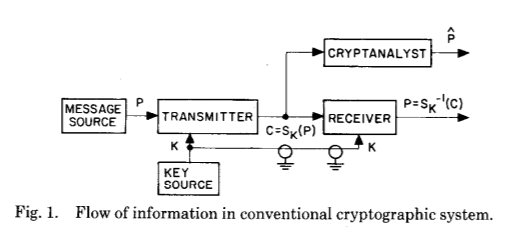And the following one: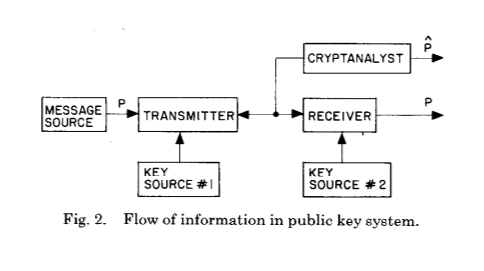These two pictures perfectly illustrate the huge change Whitfield Diffie and Martin Hellman introduced after cryptography and cryptanalysis centuries of evolution — the establishment of a shared secret key as a result of a cryptographic computation.

Let’s take a look at another good picture with colors: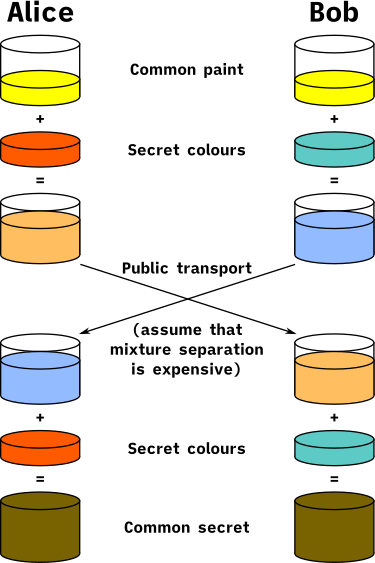It explains what is going on. Before Diffie and Hellman key agreement invention, there was only one symmetric key — it was used both to encrypt and decrypt the message. If you want to give someone such a “key”, it must be transferred over a “secure” channel. You can imagine all the restrictions of such a previous generation scheme right away — you need an already established secure channel, you cannot reuse the key, and, ideally, the length of the key should be the same as the length of the message.

Claude Shannon in his wartime classified work “Communication Theory of Secrecy Systems” proved that all theoretically unbreakable ciphers must have the same requirements as the one-time pad — famously known as the Vernam cipher, by the author of this symmetrical polyalphabetic stream cipher.

Again, we’re going to take a look at the original paper:Before we go any further, let’s ask ourselves — how two, even if brilliant, however, human beings came up with such a significant improvement in an applied field with such a history, especially at the time of war? Well, because of the:

• Information theory, formulated by Claude Shannon;
• Theory of computation influenced by, most notably, Alonzo Church, John von Neumann, and Alan Turing;
• And, more importantly, computability theory based mostly on Turing’s work, which we could say all developed and matured at the same period of the 20th century. Diffie and Hellman both mentioned Claude Shannon as the most significant influencer of their work.

Lenstra’s “Universal Security” illustrates the quantity of energy needed to “break” the symmetric cryptosystem with various key lengths. It turned out that breaking a 228-bit long, elliptic curve key would require the same amount of energy that is needed to boil all the water on Earth. It is, however, valid only under consideration of known algorithms and hardware, as, strictly speaking, no one knows if significantly more efficient algorithms or hardware exist. 228-bit EC key is comparable to the 2380-bit long RSA key, more on that later. Although in this estimation both RSA and EC keys are used in an asymmetric encryption scheme, such key lengths are somewhat equivalent to a 128-bit symmetric encryption key.

It is easy to imagine that something “hard to calculate” would require much energy and/or time needed for the computation. We tend to think that computers can “calculate everything”, but it turns out that it is not true. First, there exist undecidable examples, like the halting problem, though in the field of cryptography, we can avoid this pitfall. Second, if we consider the time needed for a particular algorithm to run, it may be arbitrary high. That is what we exploit in cryptography. A problem is considered “easy” to calculate if the time required to run the respective algorithm depends on the input size (measured in bits) like a polynomial: $$T(n) = O(n^k)$$, for some positive constant $$k$$. In the computational complexity theory field, such problems form the P complexity class.

The P complexity class is almost central, as it represents the problem for which a deterministic polynomial time algorithm exists. Another complexity class is the NP (the problems that are “hard” to calculate), representing a set of decision problems, i.e., problems requiring “yes” or “no” answer, that have a proof verifiable in polynomial time. You see the word “proof” here? That is where we get to the trapdoor functions, belonging to the NP complexity class.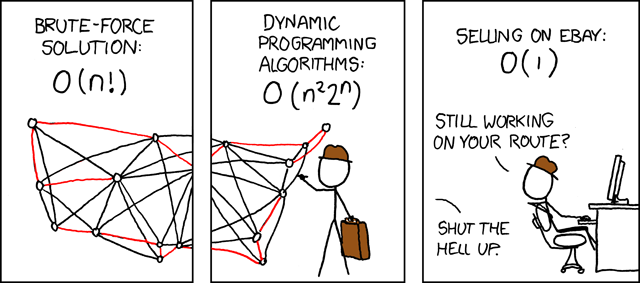Credits: xkcd

### One-way functions; Trapdoor functions

By definition, a one-way function is such a function that is easy to compute on every input but is hard to reverse, i.e. compute original input given output only. “Easy” and “hard” refer to the computational complexity theory definitions above. Interestingly, that one-way functions existence is not (mathematically) proved because their existence would prove that the P and NP complexity classes are not equal, while either P equal NP or not is nowadays an open problem. So, keep in mind that all modern cryptography relies on unproven hypotheses.

Now, in their original paper Diffie and Hellman introduce another generation of the one-way functions they called “trapdoor functions”. How do they differ?

As they explain in their landmark paper:

In a public-key cryptosystem enciphering and deciphering are governed by distinct keys, E and D, such that computing D from E is computationally infeasible (e.g. requiring $$10^{100}$$ instructions). The enciphering key E can be disclosed [in a directory] without compromising the deciphering key D. This enables any user of the system to send a message to any other user enciphered in such a way that only the intended recipient is able to decipher it ...The problem of authentication is perhaps an even more serious barrier to the universal adoption of telecommunications for business transactions than the problems of key distribution…[it]…is at the heart of any system involving contracts and billing.

By convention, cryptography characters “Alice” and “Bob” (seeking secure communication) are frequently used to explain the public-key concept. Alice and Bob agree on large integers $$n$$ and $$g$$ with $$1< g< n$$. The selection impacts the security of the system. “The modulus $$n$$ should be a prime; more importantly $$(n-1)/2$$ should also be a prime <…> and $$g$$ should be a primitive root modulo $$n$$ <…> [and] $$n$$ should be <…> at least 512 bits long.” The Diffie–Hellman protocol can be stated in elemental form in 5 steps.

1. Alice chooses $$x$$ (a large random integer) and computes $$X=g^x \bmod n$$
2. Bob chooses $$y$$ (a large random integer) and computes $$Y=g^y \bmod n$$
3. Alice sends $$X$$ to Bob, while Bob sends $$Y$$ to Alice (they keep $$x$$ and $$y$$ secret from each other)
4. Alice computes $$k = Y^x \bmod n$$
5. Bob computes $$k' = X^y \bmod n$$

As a result Alice and Bob have the same value $$k=k'$$ that serves as a shared secret.

Trapdoor function is a one-way function that makes it possible to find its inverse if one has a piece of special information called the “trapdoor”. Sounds easy, though it was rather hard to find such functions — the first feasible method was found in the implementation of a public-key cryptography asymmetric encryption algorithm named RSA after its creators: Ron Rivest, Adi Shamir and Leonard Adleman.

### RSA

In RSA the hardness of inverting the function is based on the fact that factoring (finding prime multipliers of a number) takes much more time than multiplication, or should we say here that no polynomial-time method for factoring large integers on a classical computer has been found, however, it has not been proven that none exists.

In RSA, like in any other public-key encryption system, there are two keys: public and private. RSA takes the input message (represented as a bit string) and applies a mathematical operation (exponentiation modulo a big integer) to it to get a result looking indistinguishable from random. Decryption takes this result and applies a similar operation to get back the original message. In asymmetric cryptography, the encryption is made with the public key and decryption with the private one.

How? Because the operands belong to a finite cyclic group (a set of integers with multiplication in modular arithmetic). Сomputers don’t deal great with arbitrarily big numbers, but, luckily, our cyclic group of integers is to perform an operation called “wrap around” — a number larger than the maximum allowed is wrapped around to a number in the valid range we operate. This allows us to operate with keys of “no longer than” length. In elliptic curve cryptography cyclic (multiplicative) groups are used too but constructed a bit differently as we will see later.

Basically what RSA does is taking two large prime numbers and multiplying them to get the so-called modulus. All the other numbers to be dealt with reside between zero and the modulus. The modulus is to be a part of the public key, and its bit length determines the key length. The second part of the public key is a number chosen between zero and the Euler’s totient (modern RSA implementation takes the Carmichael’s totient instead of Euler’s) of the modulus with some additional restrictions. Finally, the private key is to be calculated by solving some modular equation. To encrypt a number we simply raise it to the power equal to the public key, and to decrypt a number back, we raise it to the power equal to the private key. Thanks to the cyclic nature of the group, we get back the initial number.

There are two significant problems with the RSA nowadays, one being a consequence of the other. As the length of a key (i.e., the number of its bits) grows, the complexity factor grows not so fast as one may expect. That is because there exists sub-exponential (but still super-polynomial) factorisation algorithm. So, in order to keep an appropriate security level, the length of the RSA key needs to grow somewhat faster than the ECC key length. That is why most widespread RSA keys nowadays are either 2048 or 3072 bit long.

A bit later, we will see in numbers how the length of the key affects overall cryptosystem efficiency by comparing the RSA and ECDSA certificate signed with Let’s Encrypt authority.

### (Elliptic Curve) Digital Signature Algorithm

The search for a better trapdoor function eventually led cryptographers to an actively evolving in the mid-80s branch of mathematics dedicated to elliptic curves.

It would be the ultimate task to describe elliptic curve cryptography in one article, so we shall not. Instead, let's take a look at an elliptic curve trapdoor function based on the discrete logarithm problem.

There are many primers and more in-depth introductions into elliptic curve cryptography, and we would especially recommend Andrea Corbellini’s “ECC: a gentle introduction” if you are interested in math.

What we are interested in are rather “simple” parameters. The elliptic curve is defined by an equation like this: $$y^2 = x^3 + ax + b$$

Such a curve is needed to construct a cyclic subgroup over a finite field. Therefore, the following parameters are being used:

• The prime $$p$$ that specifies the size of the finite field;
• The coefficients $$a$$ and $$b$$ of the elliptic curve equation;
• The base point $$G$$ that generates the mentioned subgroup;
• The order $$n$$ of the subgroup;
• The cofactor $$h$$ of the subgroup.

In conclusion, the domain parameters for our algorithms is the sextuplet $$(p, a, b, G, n, h)$$.

Such elliptic curves work over the finite field $$\mathbb{F}_p$$, where $$p$$ is a rather large prime number. So we have a set of integers modulo $$p$$, where operations, such as addition, subtraction, multiplication, additive inverse, multiplicative inverse are possible. Addition and multiplication work similarly to the modular or so-called “clock” arithmetic we saw in the RSA “wrap arounds”.

Since the curve is symmetrical about the x-axis, given any point $$P$$, we can take $$−P$$ to be the point opposite it. We take $$−O$$ to be just $$O$$.

Addition for curve points is defined in a way that given points $$P$$ and $$Q$$, we can draw line intersecting both those points and intersecting curve in a third point $$R$$ so that $$P+Q=-R$$ and $$P+Q+R=0$$.

Let’s take a look at Marc Hughes explanation: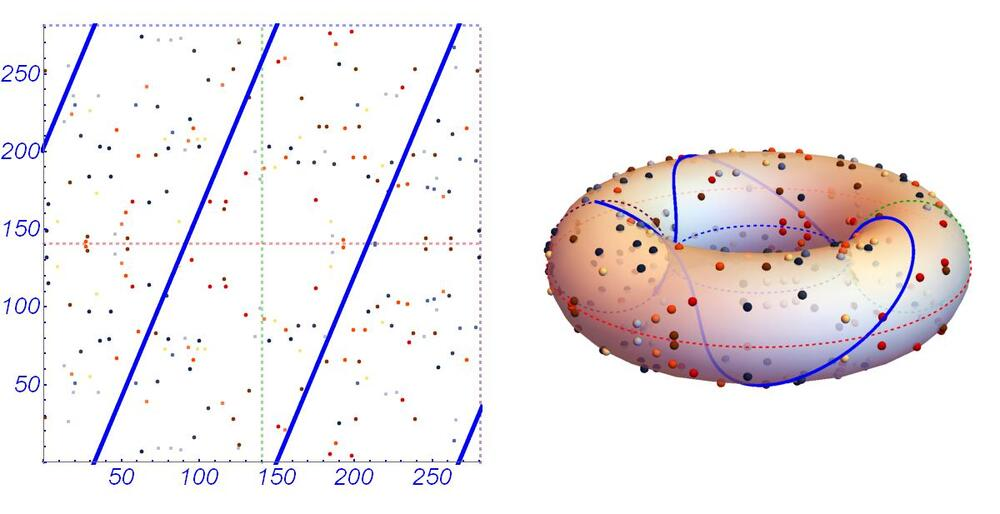A line of constant slope that travels along the surface of the torus is shown above. This line passes through two randomly selected integer points on the curve.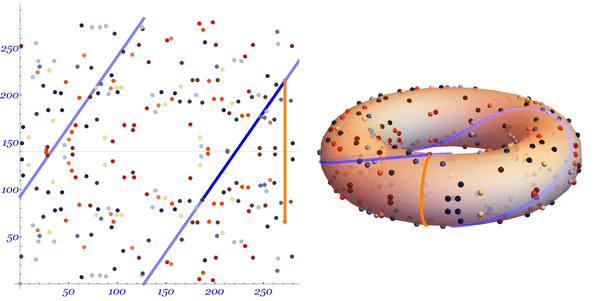To add two points on the graph, draw a line from the first selected point $$P$$ to the second selected point $$Q$$, and extend the line until it intersects another point on the graph $$-R$$, extending it across the plot boundaries if necessary.
Once you intercept an integer point, reflect the point vertically across the middle of the graph (an orange dotted line) to find the new point $$R$$ on the graph. Therefore $$P+Q=R$$.

Multiplication by a scalar is now trivial: $$n\cdot P = P + P + P + \dots + P$$ (here are $$n$$ summands).

The trapdoor function here lies within the discrete logarithm (for elliptic curves) problem, not the factorisation we took a look at within the RSA section. The problem is: if we know $$P$$ and $$Q$$, what is such $$k$$, that $$Q=k\cdot P$$?

Both the factorisation problem (underlying the RSA) and the discrete logarithm for elliptic curves (which is the basis for ECDSA and ECDH) are supposed to be hard, i.e., there are no known algorithms for solving this problem in polynomial time for a given key length.

While, typically, anyone would be shamed for mixing the key exchange (ECDH) with the signature (ECDSA) algorithm, we need to explain how they work together. A modern TLS certificate contains a public key, in our case, of the elliptic curve algorithm-generated key pair, usually signed by a higher-level authority. The client verifies the signature of the server and obtains the shared secret. The shared secret is used in a symmetric encryption algorithm, such as AES or ChaCha20. However, the principle remains the same: agree upon domain parameters (the sextuplet), get the key pair, where the private key is a randomly selected integer (the multiplicand from $$Q=k\cdot P$$), and the public key is a point at the curve. Signature algorithms use the base point $$G$$, which is a generator for a subgroup of large prime order $$n$$, such that $$n\cdot G=0$$, where 0 is the identity element. The signature proves that the secure connection is being established with the authentic party — a server which has the TLS certificate (public key) signed by some certificate authority for the given server name.

### (EC)DH(E)+ECDSA= Current handshake form

In modern TLS (1.3) the client and the server generate their public-private key pair on the fly, while establishing the connection, this is called Ephemeral version of key exchange. Most popular browser TLS libraries support this. Mostly they use Edwards 25519 elliptic curve, introduced by Daniel J. Bernstein (djb), offering 128-bit security. Since 2014 openssh uses this curve for the key pair creation. In 2019 though, browsers still don’t support TLS sessions with servers having a certificate with EdDSA public key.

But let’s get back to how things work at the end of 2019 with TLS 1.3.

The key exchange mechanisms in TLS 1.3 are restricted to (EC)DH(E)-based (with the x25519 is the one supported in client-side TLS libraries of most popular browsers as well as server-side TLS libraries, such as OpenSSL, which we will inspect a bit later), and the cipher suite list contains only three entries: TLS_AES_128_GCM_SHA256, TLS_AES_256_GCM_SHA384 and TLS_CHACHA20_POLY1305_SHA256. For those of you aware of how the cipher suites were named in the TLS 1.2 version it is apparent right away that the key exchange mechanism is now “detached” from the cipher suite name, also the static RSA and static Diffie–Hellman exchange modes removed from the specification entirely. Even the session resumption based on PSK is made over ECDHE in TLS 1.3. It is also true for custom DH parameters, which aren’t allowed now, leaving only those generally agreed to be secure in the final protocol specification.

It is interesting that there is a rather significant difference in how asymmetric encryption algorithms work nowadays. With ECC (and ECDSA certificates in particular) we use smaller keys to get to “convenient” levels of security, compared with RSA. That enables usage of a stronger asymmetric encryption algorithm and key exchange mechanisms on smaller devices and sometimes even in things that are not generally considered a device (smartcard).

First of all, it is necessary to mention what “hybrid cryptosystem” means in terms of TLS 1.3. A hybrid cryptosystem is the one that uses asymmetric (public key) encryption to establish a shared secret, that is further used as a key in a symmetric stream or block cipher.

Secondly, public-key infrastructure and certificates. It is interesting that in his 2004 interview Martin Hellman mentioned an “unsung hero” Loren Kohnfelder, whose MIT bachelor’s thesis introduced a tree structure of what we now know as public key infrastructure. Nevertheless, let’s roll back to certificates.

The fact that the server really has the private key is ensured by his signature, which can be verified with the server public key. And the certificate ensures that some public key belongs to a specific server. It means that you are establishing secure communication with the specific party and not with an imposter. Your bank, not a cybercriminal. In TLS 1.3 there is a significant improvement over previous negotiation format — the server signs all the information it has up to this moment: the client hello and the server hello, including negotiated cipher. Let’s take a look at the corresponding section of the RFC 8446:

       Client                                           Server

Key  ^ ClientHello
Exch | + key_share*
| + signature_algorithms*
| + psk_key_exchange_modes*
v + pre_shared_key*       -------->
ServerHello  ^ Key
+ key_share*  | Exch
+ pre_shared_key*  v
{EncryptedExtensions}  ^  Server
{CertificateRequest*}  v  Params
{Certificate*}  ^
{CertificateVerify*}  | Auth
{Finished}  v
<--------  [Application Data*]
^ {Certificate*}
Auth | {CertificateVerify*}
v {Finished}              -------->
[Application Data]      <------->  [Application Data]


In TLS 1.3 client sends the key share (along with needed parameters), signature algorithms right away in the first message (Client Hello). The keys needed to exchange with the server are created in the background, without the user even noticing that fact. They are further exchanged with the server to create a common secret, from pre-master secret keys that were established when the server sent his message (Server Hello) answering the client.

On the “Server Hello” side you can see the Certificate* being transferred to the client, along with the CertificateVerify* part which verifies that the party possesses the private key for the corresponding public key entry, and creates the session (symmetric) key if everything goes as planned — meaning, that the side requesting the data (client) successfully verified the answering side (server), further creating a common secret.

There are two essential operations hidden in this transmissions — signature creation and signature verification. Those are made on both sides of communication since “signature” is essentially a proof that the party actually has the private key corresponding with the public key, that the data comes from signatory and the message was not altered in transit.

With RSA, as we will further see, the signing operation is the most expensive. Since we’re signing with a 2048 or 3072-bit long key, such operation loads the server significantly, much more than it loads the client verifying such a signature.

With ECDSA, we have smaller keys (we are going to look at the ECDSA with NIST P-256 (or the secp256v1)) but more complex operations. As a result, it could be viewed as the “upside-down” RSA — the client is loaded most, by the signature verification computation, while the server easily handles the signature creation. The measurements verify that, see “A bit of benchmark” section.

This effect handily scales the nowadays Internet — since modern clients are almost equally powerful as the servers (taking in mind just the CPU core frequency), so they could effectively take the expensive operation to bare. The server, in its turn, can use the freed capabilities to create more signatures and establish more sessions.

### Let’s Encrypt certificate signature

So, in order to provide the reader with a bit of practical and handy instructions on how to create a TLS-enabled server with the ECDSA key pair signed by the Let’s Encrypt authority, we decided to illustrate a full process of creating a key pair needed to create a CSR (certificate signing request) for the Let’s Encrypt and, as a result, get the needed ECDSA certificate for our server.

We have to generate a private key to proceed. We will use the OpenSSL library. The OpenSSL manual describes the generation of any EC keys through a special command, designated especially to the elliptic curve branch of the generation algorithm.

openssl ecparam -genkey -name -secp256v1 -out privatekey.pem

To check that the OpenSSL library did everything right, we can execute the ec command.

openssl ec -in privatekey.pem -noout -text

The output will show us the specified curve the key has been created with.

Next step is quite essential to the creation of the CSR — in order to skip the process of filling all the information details needed to obtain the certificate we need the config file. Luckily, Mozilla did the entire work for us, introducing the “SSL Configuration Generator”. There, you can choose from any available server options. The pure OpenSSL config, peculiarly not present at the Generator’s page would look something like this:

[ req ]
prompt = no
encrypt_key = no
default_md = sha256
distinguished_name = dname
req_extensions = reqext

[ dname ]
CN = example.com

[ reqext ]

subjectAltName = DNS:example.com, DNS:*.example.com


Note: it is not necessary to have the CNF — if you don’t, you would be asked to fill these details in the command line.

Now, follow the creation of a CSR itself. Here we have a handy OpenSSL command.

openssl req -new -config -pathtoconfigfile.cnf -key privatekey.pem -out csr.pem

We can also verify the correctness of a newly created CSR.

openssl req -in csr.pem -noout -text -verify

Here we’ve come to a final phase — using an ACME client, certbot, to pass our certificate signing request to Let’s Encrypt.

Certbot helps you to get the needed certificate and has a whole lot of options. Here said, if you are new to the public-key encryption, and the PKI infrastructure we have in 2019, you better use --dry-run before you try to obtain the certificate for any domain of yours.

certbot certonly --dry-run --dns-somednsprovider --domain “example.com” --domain “*.example.com” --csr csr.pem

In this case, the certbot client checks that the requested domains list (in the command line) matches the domains listed in the certificate signing request. In the --dns-somednsprovider command lies a bit of a lie, because there are plenty of ways you could prove Let’s Encrypt you are in possession of a particular part of the Internet traffic. However, if you are using some public cloud hosting provider, say DigitalOcean, Hetzner, Amazon, Azure, anything — there probably would be a more natural way to provide the needed information, because your provider already made some integration tool.

After, if you are sure about the correctness of the parameters you are using in passing your CSR to the Let’s Encrypt via a certbot client — exclude the --dry-run parameter out of your command and proceed.

If successful, the client would produce several certificates as output: the signed certificate itself, the root and intermediate certs, and the combination of the latter as the last-named certificate chain, all in the .pem file format.

OpenSSL has a command we could use to inspect the certificates:

openssl x509 -in chainfilepath.pem -noout -text

At this point, it becomes evident that Let’s Encrypt signed the certificate using SHA256 digest. In addition, ECDSA Root and Intermediates signing fall under the “Upcoming Features” section, which effectively means that right now you’d get only RSA intermediates. But that’s okay since you are still using the ECDSA public key.

At the end of this section, we’d like to say something in connection to the length of keys. In information security, it is common to say the security level is 2^x, where x is the bit length (RSA is a bit of an exception here, as it grows somewhat slower than exponentially). To approximate how keys used for different algorithms correspond to each other, we would refer to the OpenSSL wiki page.

Symmetric Key Length RSA Key Length Elliptic Curve Key Length
80 1024 160
112 2048 224
128 3072 256
192 7680 384
256 15360 512

As you can see, the differences are quite prominent. Although with Let’s Encrypt we could not get any certificates signed outside of the 256 (secp256v1) and 384 (secp384r1) elliptic curve keys.

### Known issues and exceptions, and THE NSA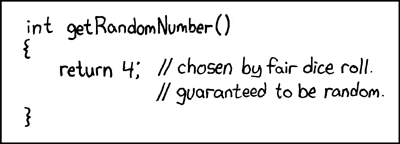Credits: xkcd

Probably, the central issue of using elliptic curve cryptography through the years was the need for a very carefully crafted random number generator, in order to create keys of required security level.

There was a massive scandal around the Dual_EC_DRBG (dual elliptic curve deterministic random bit generator) algorithm, which took many years to resolve. Also, there is uncertainty around ECC patents, as it is known that many of them belonged to the Certicom company, which was acquired by Blackberry. There also are companies that are known to be certified the use of ECC from Blackberry. Of course, there is a simple distrust in some NIST standards, which could or could not be affected by the NSA or any other United States enforcement and surveillance institution.

The implementation side of an issue is an entirely different question. In 2010 PlayStation 3 console suffered a Sony private key recovery due to improper implementation of the ECDSA algorithm —they had a static random that made the trapdoor function solvable. OpenSSL also suffered in the following year, however, quickly fixing the vulnerability that allowed retrieval of a private key with the help of a timing attack, for more information look at the original paper.

In 2013 at the RSA conference a group of researchers presented their “Randomly Failed!” paper about SecureRandom Java class vulnerabilities. Half a year later it came down to Android Bitcoin wallets, created using not enough cryptographically secure PRNG.

Due to serious serial vulnerabilities discovered, in the same August 2013, IETF released an RFC 6979, describing a deterministic generation of k used in the key creation. We could say that such a measure fixed the issue, but we won’t — anytime researchers could find issues in numerous implementations due to unnecessary deviation from the protocol specifications.

About the NSA. If you haven’t heard about the Dual_EC_DRBG story — reserve some time and read corresponding articles, you won’t regret getting into details. Edward Snowden is a part of this story because the 2013 revelations proved the earlier suspicions. It resulted in many prominent cryptographers losing trust to NIST since that organization designed and described many of the curves and further algorithms, underlying ECDSA.

Daniel Bernstein’s 25519 curve and DH function is the answer to both these issues and, as we described earlier, a transition towards EdDSA is slow though evident. Even with the NIST curves, no evidence of their vulnerability has been found yet and, as we have already mentioned, random-related experience has been quite instructive.

To conclude this part, we want to give John von Neumann’s quote: “Anyone who attempts to generate random numbers by deterministic means is, of course, living in a state of sin.”

### A bit of benchmark

We used an NGINX 1.16.0 server with OpenSSL 1.1.1d to run these benchmarks with various certificates. As we mentioned earlier, currently Let’s Encrypt allows only prime256v1 and secp384r1 algorithms for certificate signing requests, and does not provide root and intermediary ECDSA certificates, probably working at this feature at the time of us writing this article.

Signature type Handshakes per minute Handshakes per second
ECDSA (prime256v1/nistp256) 201516 3358.600
RSA 2048 58354 972.567

As you can see, for a single core of the Intel(R) Xeon(R) Silver 4114 CPU @ 2.20GHz (launched Q3’17), the overall difference in ECDSA performance, compared to the widely adopted RSA 2048 is 3.5x. Now let’s take a look at the same processor’s OpenSSL -speed results with ECDSA and RSA.

 Signature type RSA 2048 bit 256 bit ECDSA (nistp256) sign verify sign/sec verify/sec 0.000717s 0.000020s 1393.9 49458.2 0.0000s 0.0001s 38971.6 12227.1

Here we can see a confirmation for the earlier given thesis of different computational costs for the sign and verify operations of ECC and RSA. As a result, currently equipped with TLS 1.3 ECC provides a significant performance boost at the higher bit security level, compared with RSA. That is the most substantial reason why we at Qrator Labs encourage our clients to adopt ECDSA. With modern CPUs, you get almost the 5x difference in favour of ECDSA. If you are interested in how your CPU performs cryptographic computations you may run a simple openssl speed command. The -rsa, -ecdsa and -eddsa parameters would give you benchmark results for the corresponding signature algorithms.

### (Superpositioned) Future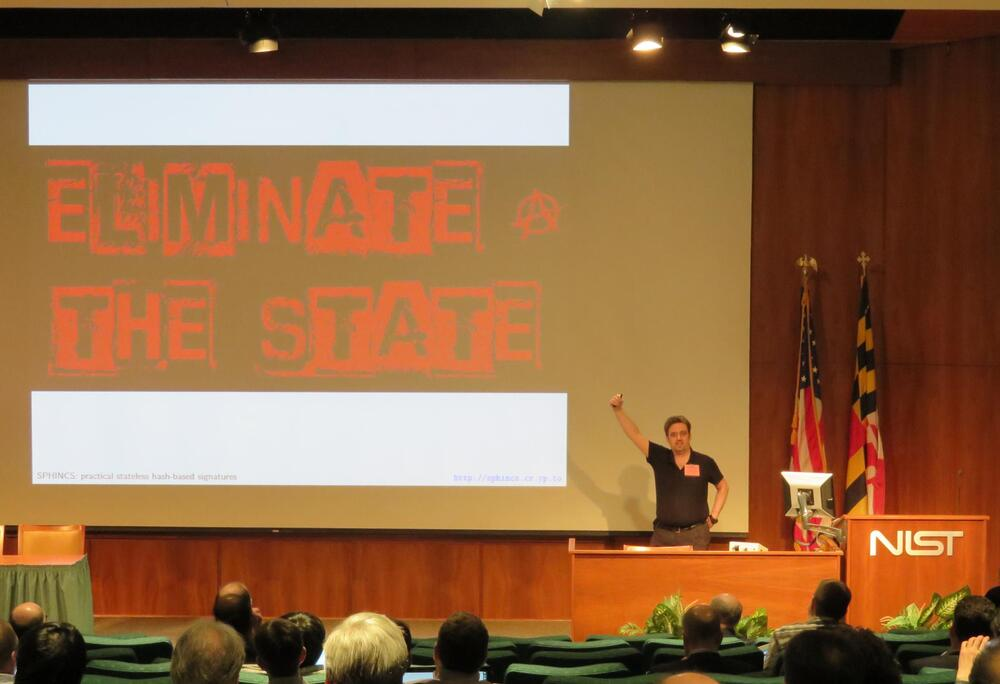Credits: djb

It is ironical that while we were preparing this article, Google announced “reaching quantum supremacy”. Does this mean that we’re in danger right now and everything developed up to this moment does not provide any secrecy?

Well, no.

As Bruce Schneier wrote in his essay for IEEE Security and Privacy “Cryptography after the Aliens Lands” a huge blow with powerful enough quantum computer could be done to the public-key (asymmetric) cryptography. Symmetric cryptography would still be strong. We want to quote Bruce Schneier with following:

There's one more future scenario to consider, one that doesn't require a quantum computer. While there are several mathematical theories that underpin the one-wayness we use in cryptography, proving the validity of those theories is in fact one of the great open problems in computer science. Just as it is possible for a smart cryptographer to find a new trick that makes it easier to break a particular algorithm, we might imagine aliens with sufficient mathematical theory to break all encryption algorithms. To us, today, this is ridiculous. Public-key cryptography is all number theory, and potentially vulnerable to more mathematically inclined aliens. Symmetric cryptography is so much nonlinear muddle, so easy to make more complex, and so easy to increase key length, that this future is unimaginable. Consider an AES variant with a 512-bit block and key size, and 128 rounds. Unless mathematics is fundamentally different than our current understanding, that'll be secure until computers are made of something other than matter and occupy something other than space.

But if the unimaginable happens, that would leave us with cryptography based solely on information theory: one-time pads and their variants.

This is the area where, except looking for implementation flaws, most issues could be found. If there is a group of well-funded mathematicians, cryptanalysts/cryptographers and computer engineers working on proving or disproving some extraordinary complex mathematical problems (like the P?=NP) and achieving substantial results up to this moment, we could be in trouble. However, such advances in computer science, information and computability theories are unlikely to be hidden, since that fact would write the names of their creators on the pages of History and, specifically, History of the Internet textbooks, which is priceless for anyone that smart. So, such a scenario could be taken out as nearly impossible.

It is not clear, whether in the nearest 5 years there would be any successes with quantum computing, though there already are several cryptography primitives considered suitable for the post-quantum world: lattices, supersingular elliptic curve isogeny-based, hashes and codes. For now, security specialists are just experimenting with them. However, there is no doubt that in the case of a need, humanity would quickly employ such algorithms at a mass scale.

For now, elliptic-curve based cryptography seems to be a perfect fit for the future decade, providing security and performance.